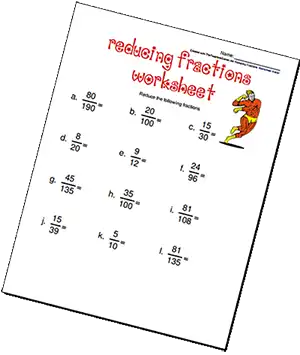# Reducing Fractions Worksheets

This Reducing Fractions Worksheet Maker will generate a series of fractions that can be reduced and you can print it out.You can customize your worksheet by choosing the number of fractions to use, the numerator and denominator range, whole numbers, improper fractions and more. When you have made your choices, simply press "Generate Worksheet", and your worksheet will open in a new window.

• max 100
• (NOTE: Numerator and denominator are for the 'reduced fraction')
to
• to

• NOTE: this is what we will multiply the fractions by created from the numbers entered above(in the background)
Example: random "reduced" fraction generated from Numerator and Denominator range above: 2/11 - we then multiply this by 2 or 3 (multiplier) to give your student a fraction to reduce. So we would show them 4/22 or 6/33
to
Choose up to 3 fonts + Arial (default)
120+ Fonts below are for Premium Members
NOTE: Certain fonts may not have all punctuation